# 3.7 Derivatives of inverse functions  (Page 3/3)

 Page 3 / 3

Find the derivative of $h\left(x\right)={\text{cos}}^{-1}\left(3x-1\right).$

${h}^{\prime }\left(x\right)=\frac{-3}{\sqrt{6x-9{x}^{2}}}$

## Applying the inverse tangent function

The position of a particle at time $t$ is given by $s\left(t\right)={\text{tan}}^{-1}\left(\frac{1}{t}\right)$ for $t\ge \frac{1}{2}.$ Find the velocity of the particle at time $t=1.$

Begin by differentiating $s\left(t\right)$ in order to find $v\left(t\right).$ Thus,

$v\left(t\right)={s}^{\prime }\left(t\right)=\frac{1}{1+{\left(\frac{1}{t}\right)}^{2}}·\frac{-1}{{t}^{2}}.$

Simplifying, we have

$v\left(t\right)=-\frac{1}{{t}^{2}+1}.$

Thus, $v\left(1\right)=-\frac{1}{2}.$

Find the equation of the line tangent to the graph of $f\left(x\right)={\text{sin}}^{-1}x$ at $x=0.$

$y=x$

## Key concepts

• The inverse function theorem allows us to compute derivatives of inverse functions without using the limit definition of the derivative.
• We can use the inverse function theorem to develop differentiation formulas for the inverse trigonometric functions.

## Key equations

• Inverse function theorem
${\left({f}^{-1}\right)}^{\prime }\left(x\right)=\frac{1}{{f}^{\prime }\left({f}^{-1}\left(x\right)\right)}$ whenever ${f}^{\prime }\left({f}^{-1}\left(x\right)\right)\ne 0$ and $f\left(x\right)$ is differentiable.
• Power rule with rational exponents
$\frac{d}{dx}\left({x}^{m\text{/}n}\right)=\frac{m}{n}{x}^{\left(m\text{/}n\right)-1}.$
• Derivative of inverse sine function
$\frac{d}{dx}\phantom{\rule{0.1em}{0ex}}{\text{sin}}^{-1}x=\frac{1}{\sqrt{1-{\left(x\right)}^{2}}}$
• Derivative of inverse cosine function
$\frac{d}{dx}\phantom{\rule{0.1em}{0ex}}{\text{cos}}^{-1}x=\frac{-1}{\sqrt{1-{\left(x\right)}^{2}}}$
• Derivative of inverse tangent function
$\frac{d}{dx}\phantom{\rule{0.1em}{0ex}}{\text{tan}}^{-1}x=\frac{1}{1+{\left(x\right)}^{2}}$
• Derivative of inverse cotangent function
$\frac{d}{dx}\phantom{\rule{0.1em}{0ex}}{\text{cot}}^{-1}x=\frac{-1}{1+{\left(x\right)}^{2}}$
• Derivative of inverse secant function
$\frac{d}{dx}\phantom{\rule{0.1em}{0ex}}{\text{sec}}^{-1}x=\frac{1}{|x|\sqrt{{\left(x\right)}^{2}-1}}$
• Derivative of inverse cosecant function
$\frac{d}{dx}\phantom{\rule{0.1em}{0ex}}{\text{csc}}^{-1}x=\frac{-1}{|x|\sqrt{{\left(x\right)}^{2}-1}}$

For the following exercises, use the graph of $y=f\left(x\right)$ to

1. sketch the graph of $y={f}^{-1}\left(x\right),$ and
2. use part a. to estimate ${\left({f}^{-1}\right)}^{\prime }\left(1\right).$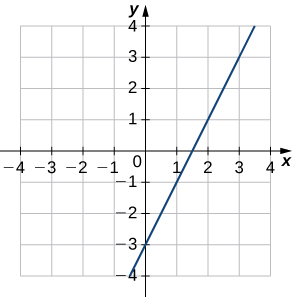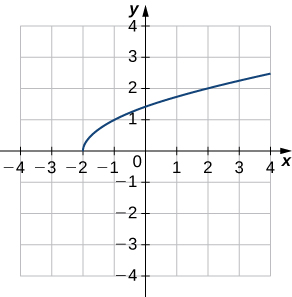a.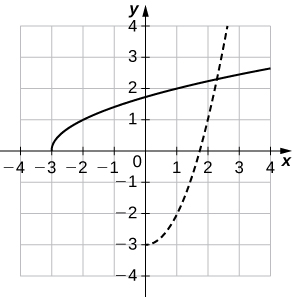b. ${\left({f}^{-1}\right)}^{\prime }\left(1\right)~2$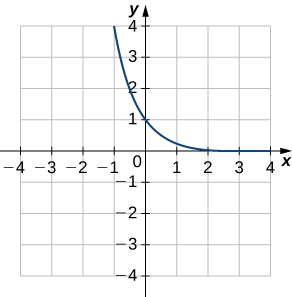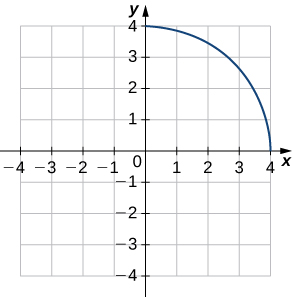a.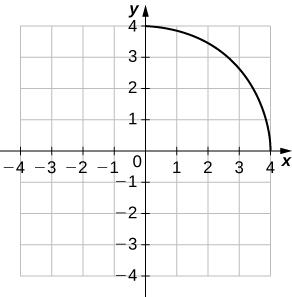b. ${\left({f}^{-1}\right)}^{\prime }\left(1\right)~-1\text{/}\sqrt{3}$

For the following exercises, use the functions $y=f\left(x\right)$ to find

1. $\frac{df}{dx}$ at $x=a$ and
2. $x={f}^{-1}\left(y\right).$
3. Then use part b. to find $\frac{d{f}^{-1}}{dy}$ at $y=f\left(a\right).$

$f\left(x\right)=6x-1,x=-2$

$f\left(x\right)=2{x}^{3}-3,x=1$

a. 6, b. $x={f}^{-1}\left(y\right)={\left(\frac{y+3}{2}\right)}^{1\text{/}3},$ c. $\frac{1}{6}$

$f\left(x\right)=9-{x}^{2},0\le x\le 3,x=2$

$f\left(x\right)=\text{sin}\phantom{\rule{0.1em}{0ex}}x,x=0$

a. $1,$ b. $x={f}^{-1}\left(y\right)={\text{sin}}^{-1}y,$ c. $1$

For each of the following functions, find ${\left({f}^{-1}\right)}^{\prime }\left(a\right).$

$f\left(x\right)={x}^{2}+3x+2,x\ge -1,a=2$

$f\left(x\right)={x}^{3}+2x+3,a=0$

$\frac{1}{5}$

$f\left(x\right)=x+\sqrt{x},a=2$

$f\left(x\right)=x-\frac{2}{x},x<0,a=1$

$\frac{1}{3}$

$f\left(x\right)=x+\text{sin}\phantom{\rule{0.1em}{0ex}}x,a=0$

$f\left(x\right)=\text{tan}\phantom{\rule{0.1em}{0ex}}x+3{x}^{2},a=0$

$1$

For each of the given functions $y=f\left(x\right),$

1. find the slope of the tangent line to its inverse function ${f}^{-1}$ at the indicated point $P,$ and
2. find the equation of the tangent line to the graph of ${f}^{-1}$ at the indicated point.

$f\left(x\right)=\frac{4}{1+{x}^{2}},P\left(2,1\right)$

$f\left(x\right)=\sqrt{x-4},P\left(2,8\right)$

a. $4,$ b. $y=4x$

$f\left(x\right)={\left({x}^{3}+1\right)}^{4},P\left(16,1\right)$

$f\left(x\right)=\text{−}{x}^{3}-x+2,P\left(-8,2\right)$

a. $-\frac{1}{96},$ b. $y=-\frac{1}{13}x+\frac{18}{13}$

$f\left(x\right)={x}^{5}+3{x}^{3}-4x-8,P\left(-8,1\right)$

For the following exercises, find $\frac{dy}{dx}$ for the given function.

$y={\text{sin}}^{-1}\left({x}^{2}\right)$

$\frac{2x}{\sqrt{1-{x}^{4}}}$

$y={\text{cos}}^{-1}\left(\sqrt{x}\right)$

$y={\text{sec}}^{-1}\left(\frac{1}{x}\right)$

$\frac{-1}{\sqrt{1-{x}^{2}}}$

$y=\sqrt{{\text{csc}}^{-1}x}$

$y={\left(1+{\text{tan}}^{-1}x\right)}^{3}$

$\frac{3{\left(1+{\text{tan}}^{-1}x\right)}^{2}}{1+{x}^{2}}$

$y={\text{cos}}^{-1}\left(2x\right)·{\text{sin}}^{-1}\left(2x\right)$

$y=\frac{1}{{\text{tan}}^{-1}\left(x\right)}$

$\frac{-1}{\left(1+{x}^{2}\right){\left({\text{tan}}^{-1}x\right)}^{2}}$

$y={\text{sec}}^{-1}\left(\text{−}x\right)$

$y={\text{cot}}^{-1}\sqrt{4-{x}^{2}}$

$\frac{x}{\left(5-{x}^{2}\right)\sqrt{4-{x}^{2}}}$

$y=x·{\text{csc}}^{-1}x$

For the following exercises, use the given values to find ${\left({f}^{-1}\right)}^{\prime }\left(a\right).$

$f\left(\pi \right)=0,f\prime \left(\pi \right)=-1,a=0$

$-1$

$f\left(6\right)=2,{f}^{\prime }\left(6\right)=\frac{1}{3},a=2$

$f\left(\frac{1}{3}\right)=-8,f{\prime }^{}\left(\frac{1}{3}\right)=2,a=-8$

$\frac{1}{2}$

$f\left(\sqrt{3}\right)=\frac{1}{2},f{\prime }^{}\left(\sqrt{3}\right)=\frac{2}{3},a=\frac{1}{2}$

$f\left(1\right)=-3,f\prime \left(1\right)=10,a=-3$

$\frac{1}{10}$

$f\left(1\right)=0,f{\prime }^{}\left(1\right)=-2,a=0$

[T] The position of a moving hockey puck after $t$ seconds is $s\left(t\right)={\text{tan}}^{-1}t$ where $s$ is in meters.

1. Find the velocity of the hockey puck at any time $t.$
2. Find the acceleration of the puck at any time $t.$
3. Evaluate a. and b. for $t=2,4,$ and $6$ seconds.
4. What conclusion can be drawn from the results in c.?

a. $v\left(t\right)=\frac{1}{1+{t}^{2}}$ b. $a\left(t\right)=\frac{-2t}{{\left(1+{t}^{2}\right)}^{2}}$ c. $\left(a\right)0.2,0.06,0.03;\left(b\right)-0.16,-0.028,-0.0088$ d. The hockey puck is decelerating/slowing down at 2, 4, and 6 seconds.

[T] A building that is 225 feet tall casts a shadow of various lengths $x$ as the day goes by. An angle of elevation $\theta$ is formed by lines from the top and bottom of the building to the tip of the shadow, as seen in the following figure. Find the rate of change of the angle of elevation $\frac{d\theta }{dx}$ when $x=272$ feet.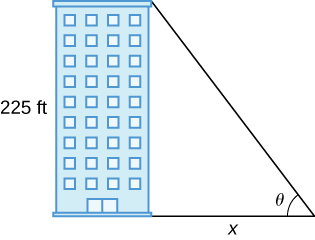[T] A pole stands 75 feet tall. An angle $\theta$ is formed when wires of various lengths of $x$ feet are attached from the ground to the top of the pole, as shown in the following figure. Find the rate of change of the angle $\frac{d\theta }{dx}$ when a wire of length 90 feet is attached.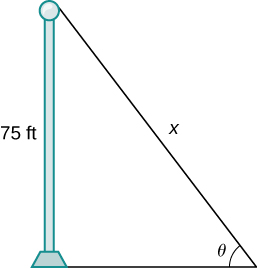$-0.0168$ radians per foot

[T] A television camera at ground level is 2000 feet away from the launching pad of a space rocket that is set to take off vertically, as seen in the following figure. The angle of elevation of the camera can be found by $\theta ={\text{tan}}^{-1}\left(\frac{x}{2000}\right),$ where $x$ is the height of the rocket. Find the rate of change of the angle of elevation after launch when the camera and the rocket are 5000 feet apart.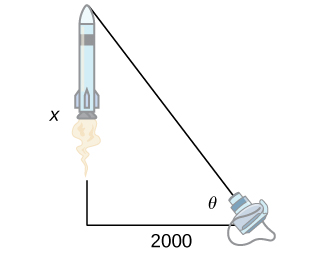[T] A local movie theater with a 30-foot-high screen that is 10 feet above a person’s eye level when seated has a viewing angle $\theta$ (in radians) given by $\theta ={\text{cot}}^{-1}\frac{x}{40}-{\text{cot}}^{-1}\frac{x}{10},$

where $x$ is the distance in feet away from the movie screen that the person is sitting, as shown in the following figure.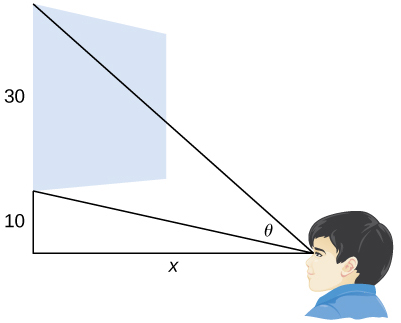1. Find $\frac{d\theta }{dx}.$
2. Evaluate $\frac{d\theta }{dx}$ for $x=5,10,15,$ and 20.
3. Interpret the results in b..
4. Evaluate $\frac{d\theta }{dx}$ for $x=25,30,35,$ and 40
5. Interpret the results in d. At what distance $x$ should the person stand to maximize his or her viewing angle?

a. $\frac{d\theta }{dx}=\frac{10}{100+{x}^{2}}-\frac{40}{1600+{x}^{2}}$ b. $\frac{18}{325},\frac{9}{340},\frac{42}{4745},0$ c. As a person moves farther away from the screen, the viewing angle is increasing, which implies that as he or she moves farther away, his or her screen vision is widening. d. $-\frac{54}{12905},-\frac{3}{500},-\frac{198}{29945},-\frac{9}{1360}$ e. As the person moves beyond 20 feet from the screen, the viewing angle is decreasing. The optimal distance the person should stand for maximizing the viewing angle is 20 feet.

how does this work
Can calculus give the answers as same as other methods give in basic classes while solving the numericals?
log tan (x/4+x/2)
Rohan
Rohan
y=(x^2 + 3x).(eipix)
Claudia
Ismael
A Function F(X)=Sinx+cosx is odd or even?
neither
David
Neither
Lovuyiso
f(x)=1/1+x^2 |=[-3,1]
apa itu?
fauzi
determine the area of the region enclosed by x²+y=1,2x-y+4=0
Hi
MP
Hi too
Vic
hello please anyone with calculus PDF should share
Which kind of pdf do you want bro?
Aftab
hi
Abdul
can I get calculus in pdf
Abdul
How to use it to slove fraction
Hello please can someone tell me the meaning of this group all about, yes I know is calculus group but yet nothing is showing up
Shodipo
You have downloaded the aplication Calculus Volume 1, tackling about lessons for (mostly) college freshmen, Calculus 1: Differential, and this group I think aims to let concerns and questions from students who want to clarify something about the subject. Well, this is what I guess so.
Jean
Im not in college but this will still help
nothing
how can we scatch a parabola graph
Ok
Endalkachew
how can I solve differentiation?
with the help of different formulas and Rules. we use formulas according to given condition or according to questions
CALCULUS
For example any questions...
CALCULUS
v=(x,y) وu=(x,y ) ∂u/∂x* ∂x/∂u +∂v/∂x*∂x/∂v=1
log tan (x/4+x/2)
Rohan
what is the procedures in solving number 1?
review of funtion role?
for the function f(x)={x^2-7x+104 x<=7 7x+55 x>7' does limx7 f(x) exist?
find dy÷dx (y^2+2 sec)^2=4(x+1)^2
Integral of e^x/(1+e^2x)tan^-1 (e^x)
why might we use the shell method instead of slicing
fg[[(45)]]²+45⅓x²=100By OpenStaxBy Jessica CollettBy Inderjeet BrarBy OpenStaxBy Richley CrapoBy Yasser IbrahimBy Mucho MizindukoBy Sandhills MLTBy OpenStaxBy Briana Hamilton